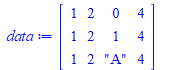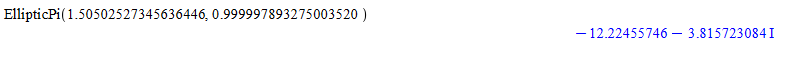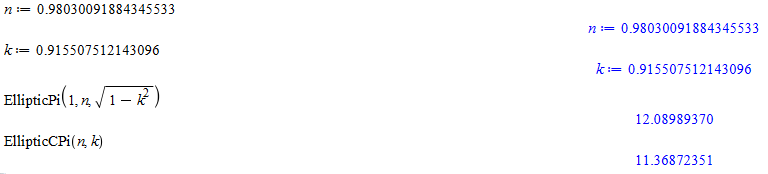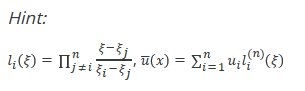## Maple soft project...

1) Without the aid of a graph, find the absolute maximum and minimum values of the function

f(x)=36x6 +2665x4 +240x−675+4534x2 −5836x3−516x5 over the interval [0,4]

2) Find points P and Q on the parabola y = 1 − x 2 so that the triangle ABC formed by the x-axis and the tangent lines at P and Q is an equilateral triangle.

## what solver PDEtools:-Solve uses by default...

I noticed PDEtools:-Solve hangs sometimes on some input where solve does not. When I change the solver for PDEtools:-Solve to use solve, it did not hang.

This means PDEtools:-Solve default solver is something else. I did not see what it is by looking at help.

Here is an example

restart;
eq:=[1/3*(-334*I*(-4807763*I+27000*I*2^(1/2)+60*(1746675+72116445*2^(1/2))^(1/2))^(1/3)-2*(-4807763*I+27000*I*2^(1/2)+60*(1746675+72116445*2^(1/2))^(1/2))^(2/3)+56978)/(-4807763*I+27000*I*2^(1/2)+60*(1746675+72116445*2^(1/2))^(1/2))^(1/3)*v-20*I*2^(1/2)*v = 0, (-18000*I*2^(1/2)+33400*I-40*(1746675+72116445*2^(1/2))^(1/2)-40*(-167*I*(1746675+72116445*2^(1/2))^(1/2)+75150*2^(1/2)+10*(-4807763*I+27000*I*2^(1/2)+60*(1746675+72116445*2^(1/2))^(1/2))^(2/3)+145445)/(-4807763*I+27000*I*2^(1/2)+60*(1746675+72116445*2^(1/2))^(1/2))^(1/3))/(167*I*(-4807763*I+27000*I*2^(1/2)+60*(1746675+72116445*2^(1/2))^(1/2))^(1/3)+(-4807763*I+27000*I*2^(1/2)+60*(1746675+72116445*2^(1/2))^(1/2))^(2/3)-28489)*v-20*I*2^(1/2)*t = 0];

solve(eq,[v,v]); #OK
PDEtools:-Solve(eq,[v,v],solver=solve); #OK
PDEtools:-Solve(eq,[v,v]); #hangs


Maple 2021

## how to use uses between child modules? ...

I am merging two separate modules  B,C to be child modules inside one main A module.

Now, I found the code breaks, because uses does not work any more.

Before merging, C module did uses B. But now this gives an error when both B,C sit inside one parent module.  An example make this easier to explain

restart;
interface(warnlevel=4);
kernelopts('assertlevel'=2):

A:=module()

export B:=module()
export foo:=proc()
print("In B:-foo()");
end proc;
end module;

export C:=module()
uses B; #this cases problem
export boo:=proc()
foo();
end proc;
end module;
end module;

Error, (in A:-C) no bindings were specified or implied


Changing uses B; to uses A:-B; does not help. I get error Error, (in A:-C) A does not evaluate to a module

I also tried uses :-A:-B; , now this does not give error, but it does not work. i.e. when doing  A:-C:-boo() Maple does not end up calling foo() inside module B as expected.

One way to avoid all this, is not to use uses B inside the module and do this instead

A:=module()

export B:=module()
export foo:=proc()
print("In B:-foo()");
end proc;
end module;

export C:=module()
export boo:=proc()
B:-foo();
end proc;
end module;
end module;

But it means I have to now change lots of code inside the C module, and add an explicit B:- everywhere

This is how it was before the merging

B:=module()
export foo:=proc()
print("In B:-foo()");
end proc;
end module;

C:=module()
uses B;    #no problem now
export boo:=proc()
foo();  #this now uses B:-foo() automatically due to uses.
end proc;
end module;


The above works. Now I can do C:-boo() and it works as expected.

The question is: How to make it work after moving both C and B inside one parent module so I do not have to change lots of code? I tried many things, but can't get it to work.

Maple 2021 on windows 10

Edit

Thanks for the answers below. I think I have to change my code then either way to add a prefix to the call. I actually always use the long form of the call for everything as in module:-function() but for one function, which I use so much everywhere, using the fully qualified name would make things hard to read.  This function converts Maple expressions to Latex after some filtering. Here is an example

cat(",toX(y),"' = f_0(",toX(f),")",toX(y),"+f_1(",toX(x),")",toX(g),"^n \\tag{2}, etc.....")

Now the function toX comes from one module, the one I had uses for it. Now I have to change the above to becomes

",module_name:-toX(f),"' = f_0(",module_name:-toX(x),")",module_name:-toX(y),"+f_1(",module_name:-toX(x),")",module_name:-toX(g),"^n \\tag{2}

Since my strings are very long as it is, (program generates Latex on the fly as it runs), this will make them even longer and harder to read.

But I can change all this in the editor, using global search and replace.

I was just hoping I do not have to just because I moved the modules all into one main module. I still do not understand why Maple does not allow uses when moving the modules inside one bigger module, but I guess this is by design.

## How to index a list by a list of indices?...

Hello

I have a couple of large lists that will be further processed.  One of the steps is to index a list using another list (a list of indices).  A short example will be something like

L:=[2,1,4,3,7,6,8,3,4,5];
ind:=[2,2,3,3,5,6,7,1,4,10];


The solution

L1:=L[ind]:

does not seem to be a good choice since it takes longer than the following solution

L1:=Threads:-Map(w->L[w],ind):

Since I am not a Maple expert, it is very likely that is a faster solution.

Many thanks

## Group assignment...

hey guys, I'm curious if there is any way to do a group assignment like the following

JointNum := 3;

seq(alpha[i], i = 1 .. JointNum) := 0, 0, 0;


which should as result be the following assignment

alpha, alpha, alpha:=0,0,0

which is a valid assignment standalone but not works in the sequence style form . how can I implement this type of assignment

## Java OpenMaple Running Error...

Hi, when I tried the simple example of Java OpenMaple on Mac, I can compile the code but couldn't run it. It complained about not finding libjopenmaple.jnilib. I checked that library directory, there was /libjopenmaple.jnilib but not libmaplec.dylib. Any suggestions?

> java -Djava.library.path=/Library/Frameworks/Maple.framework/Versions/2021/bin.APPLE_UNIVERSAL_OSX -classpath "$MAPLE/java/externalcall.jar:$MAPLE/java/Maple.jar:." test

Referenced from: /Library/Frameworks/Maple.framework/Versions/2021/bin.APPLE_UNIVERSAL_OSX/libjopenmaple.jnilib
Referenced from: /Library/Frameworks/Maple.framework/Versions/2021/bin.APPLE_UNIVERSAL_OSX/libjopenmaple.jnilib
at com.maplesoft.openmaple.Engine.<clinit>(Engine.java:23)
at test.main(test.java:22)
at com.maplesoft.openmaple.Engine.getKernel(Native Method)
at com.maplesoft.openmaple.Engine.<init>(Engine.java:44)
at test.main(test.java:22)

## ODE Plotting problem...

Dear Community,

I try to slove a simple 2nd order ODE describing a simple pulley. Unfortunately dsolve does not like what I'm trying to do, and I get en error message. My goal is to plot travel distance, velocity and acceleration (  h(t), dh(t)/dt, d2h(t)/dt2 ) of the lifted weight. Could you pls. have a look what I'm doing wrong?

Also is there a way to transform this worksheet to a MapleSim document somehow? Worksheet attached.

best regards

Andras

SimplePulley.mw

## What's With the User Interface in Maple 2021?...

Since I installed Maple 2021 I have noticed a problem with the left corner of the menu and toolbar.  See the screenshot attached. I don't know if this is a general problem in Win10 or is this a problem with my installation.## How to share a submodule among other modules insid...

Edit

I found the problem. Let me rephrase it. I keep original question below.

This causes an error

restart;
interface(warnlevel=4);
kernelopts('assertlevel'=2):
A:=module()
export module_A1;
local person_type;

module person_type()
option object;
export name::string;
export age::string;
end module;

module_A1:=module()
export boo;
boo:=proc()
local X::A:-person_type;  #THIS LINE CAUSES ERROR
#local X::person_type;    #THIS LINE ALSO CAUSES ERROR
#local X;                 #THIS WORKS. No error
X:=Object(person_type);
return X;
end proc;
end module:
end module:

A:-module_A1:-boo();
Error, (in boo) module does not export person_type


If I change  local X::person_type;  to just local X;  then no error is generated:

restart;
interface(warnlevel=4);
kernelopts('assertlevel'=2):

A:=module()
export module_A1;
local person_type;

module person_type()
option object;
export name::string;
export age::string;
end module;

module_A1:=module()
export boo;
boo:=proc()
local X;
X:=Object(person_type);  #now this works
return X;
end proc;
end module:

end module:

A:-module_A1:-boo();

Object<<person_type,1822742359104>>


So the problem was in the variable declaration. Maple for some reason does not like   local X::A:-person_type;  and does not like local X::person_type; but things seem to work by just removing the ::type altogother.

So this solves this problem for me for now, I can keep person module local, and still use it in other child modules. Even though I do not understand why Maple complains about it when I use ::

original question. Can be ignored now.

I have one main module, with 2 submodules (child modules) A1 and A2. The child modules are all exported so they can be called from outside the main module.

But I want to have one additional module X as object, to share among the child modules A1 and A2, but without having to also export module X.

But Maple wants me to also export module X in order to use it inside the child modules A1, and A2.

Is there a way to share X  among child modules, without exporting it? I only want X be visible to child modules (i.e. modules inside the main module)

The module X has option object and I want to use it as just a type basically (instead of using Record).

Here is an example

restart;
interface(warnlevel=4);
kernelopts('assertlevel'=2):
A:=module()
export module_A1,module_A2;
local person_type;

module person_type()
option object;
export name::string;
export age::string;
end module;

module_A1:=module()
export boo;
boo:=proc()
local X::A:-person_type;
X:=Object(A:-person_type);
return 1;
end proc;
end module:

module_A2:=module()
export boo;
boo:=proc()
local X::A:-person_type;
X:=Object(A:-person_type);
return 1;
end proc;
end module:

end module:



Now the call A:-module_A1:-boo(); fail with Error, (in boo) module does not export person_type

This can be fixed by changing local person_type;  to export person_type;  but this means person_type can now be seen from outside the main package.

In Ada, it is possible to have private data type shared among child packages. How to do the same in Maple?

## I used to only have to press F5 once to switch bet...

Whenever i press F5, Maple will switch to nonexecutable math so I have to press it once more to go to math. This is very annoying. I would very much like to have it just switch between math and text when i press F5 like it used to do.
How do I make it so?

## import CSV changes "1" to number. ...

I have plain text files with comma delimited fields. Some fileds are strings and some are numeric.

When importing the file using

data:=Import(file_name,format="CSV",output=Matrix);

Maple changes any field which is string but contains a number inside it, as in "1"  to number

And this causes all sorts of problems for me when processing the matrix data, since the program expects some fields to be strings.

I looked at all options, but do not see how to tell Maple to keep a string as string, even if what is inside it is a number.

Here is an example file  "t.txt"

1,2,"0",4
1,2,"1",4
1,2,"A",4

restart;
file_name:="C:/TMP/t.txt";
data:=Import(file_name,format="CSV",output=Matrix);


And Maple returnsYou see it changed "0" to 0 and "1" to 1.

This might be how CSV is supposed to work?. Does Maple have any option to override this default behavior in order to keep strings as strings, even though there is a number inside the string. I'll try to see if there is another format other than CSV to try which might behave better.

Maple 2021 on windows 10.

## easy way to remove all specific substrings from on...

I could not find a function in StringTools package which removes all substrings (not single characters) from one long string directly.

For example given a Latex string

s:=" \\left \\int x \\,dx \\left";

And I want to remove all "\\left"  from it, which results in "  \\int x \\,dx "

In Mathematica, there is a function called StringDelete which removes all occurances of the substring in one call. Like this

s = " \\left \\int x \\,dx \\left";
StringDelete[s, "\\left"]

In Maple, I tried StringTools:-Remove(x->evalb(x="\\left"),s) and this did not work.Also tried

s:=" \\left \\int x \\,dx \\left";
StringTools:-Remove(x->StringTools:-Compare(x,"\\left"),s)


But this returned "leftintxdxleft"

And StringTools:-Delete needs a range, which one then has to first find.

I found this:

s:=" \\left \\int x \\,dx \\left";
idx:=[StringTools:-SearchAll("\\left",s)]; #returns index of first character in the string
StringTools:-Delete(s,idx..idx+4); #this only remove the first one. Need to loop to remove all.


Which returns the first substring. So one has to make a loop and keep removing all substrings found from the call to StringTools:-SearchAll

This is all too much work. But can be done.

The question is: What is the simplist way to remove all substrings from one string? All substrings are the same and the substrings can be more than one character.

Here is another example

s:="1234566X6Y67890A6BC66DEFGH";

And want to remove all "66" substrings, so the result will be

"12345X6Y67890A6BCDEFGH";

I am sure there must be an easier way to do this in Maple than having to call 2 or 3 functions and using a loop, but I could not find it so far.

What would be the closest function in Maple to Mathematica's StringDelete?

## Complex value of EllipticPi...

Hi there.

Following by https://www.mapleprimes.com/questions/232199-Bug-In-EllipticCPi

there is some strange behavior of EllipticPi - this function return complex answer when nu>1WolframAlpha and some another online calculators return real values - just real part of Maple's value.

Why Maple's EllipticPi return complex answer?

## Bug in EllipticCPi...

Hi there.

It seems like EllipticCPi(n,k) has a bug when n close to 1:Both answers should be equal by definition and first is true.

ell_error.mw

## How make Maple calculate automatically the Lagrang...

Hello everybody

In a course on numerical calculation in seismology (with jupyter notebook but I tried to adapt them in Maple), we use the Lagrangian interpolation:First, have done the calculation and get the right answer. But I had to do it manually in Maple (see document below for the question and my solution. The question is describe more explicitly. What I want to do is to make Maple do it automatically and taking into account that there is the restriction i<>j. Then when the lagrangian is calculated, it will be easy to calculate u bar.